# How To Count Number Of Days Between Dates In Excel

As an Amazon Associate I earn from qualifying purchases.

Counting number of days between two different dates by yourself can be a handful sometimes. You can manage to count the days if both the dates fall on the same month. It gets trickier when the second date falls on another month or year. Nowadays there are many tools on the internet that will do that for you. But if you are working on MS Excel and you need to input dates and days between them, you can do it there itself. It doesn’t matter if the date has passed or if the date lies in the future. You can also count only the weekdays or months, years that fell in between two dates. In Excel, you can do this by various methods and we are going to explain each of them.

## Count Days Between Dates In Excel

### 1. Using A Simple Formula

If you want a quick solution to it, and only want to find out days between a few dates, you can do it by using a simple formula. Before the formula, this is what you need to do. Write both the dates in different cells and click on a cell where you need the difference to appear. Once you have done that apply the formula =newer date cell-older date cell. If you have entered the newer date in C3 and older date in C2 then the formula will be =C3-C2.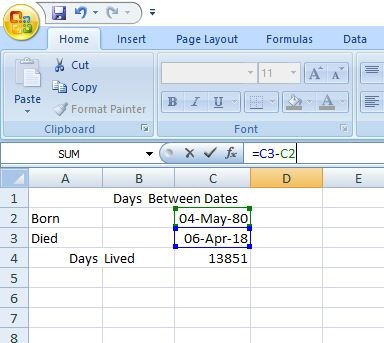Remember to subtract an older date from the newer date, otherwise, you will get the result in a negative integer.

You can also use this formula to find out the number of days between multiple pairs of dates.

Note: You don’t need to apply the formula manually for each instance. Just drag cell downward to apply the same formula in every cell automatically.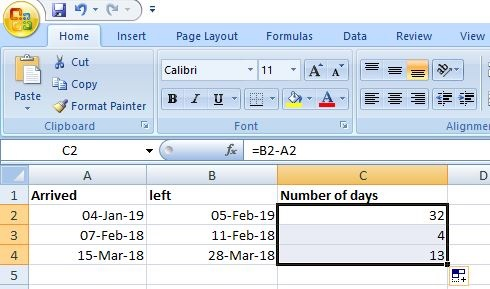### Working Of This Formula

What Microsoft Excel does is, it stores dates and assign them a serial number. The date 01-Jan-1900 is assigned the number 1, 02-Jan-1900 is assigned the number 2, 3-Jan-1900 is assigned the number 3 and so on. So instead of subtracting one date from other it actually subtracts integers and gives an accurate result. In our example, the date 04-May-1980 is assigned with the numeric value of 29343 and 06-Apr-2018 is assigned with the numeric value of 43194. Subtracting both of these numbers give the result of 13851 i.e. the difference of days between these two dates.

### 2. Calculate Days By Using DAYS Function

This is another simple way to find out days between two dates is by using DAYS function. The only exception is, it only works with users of Excel 2013 and 2016. Otherwise, it will show a #NAME? error, that is invalid name error. Once you have entered all the necessary dates, insert the formula =DAYS(end_date_cell, start_date_cell) in the function section. So if your end date lies in the cell B2 and start date lies in the cell A2, the formula will be =DAYS(B2, A2). Just like the first method, it will return days as a positive or negative integer depending on the placement of dates.

### 3. Calculate Days By Using DATEDIF Function

This function is very similar to DAYS function but different at the same time. Instead of two arguments, it has an additional argument “d” that represents days. You can also use “m” and “y” instead of “d” to find out months and years instead of days.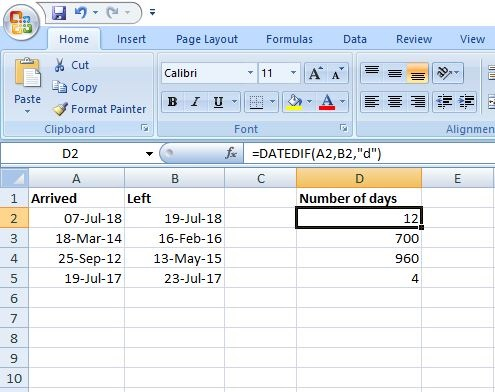The formula goes like this =DATEDIF(start_date_cell,end_date_cell, “d”). In our example, we have used =DATEDIF(A2, B2, “d”) to get the result. You might have noticed that unlike DAYS function we have mentioned start date cell in the first argument and end date cell in the second. If it is not done in this order it will return an error #NUM! instead of a negative integer like in the example below.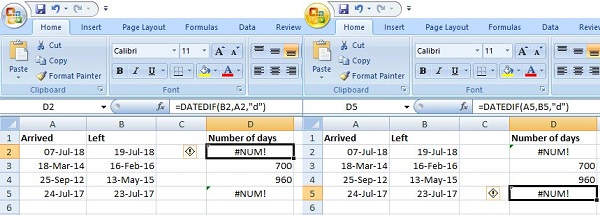You can see that it returned an error in both cases. In first I have shuffled the arguments and in second the formula the arguments are in order but the start date is smaller than the end date.  This function is useful because like I told before can also count months and years between two dates. But keep in mind that it will round-off the result to a whole number in this case. We ran this function in Excel 2007, so it can be used in older versions of MS Excel.

### 4. Calculate Number Of Days Between Today And Any Other Date

Maybe want to find out the number of days left for an event or number of days passed since anything happened. By using this method you can find out the number of days left until a future date and number of days passed since a past date. In the function section use DATE function by using this formula =DATE(future_date)-TODAY() for finding out days left between today and a future date. Likewise, use the formula =TODAY()-DATE(past_date) to find out the number of days since.

In our example, we have found out days left between today and new years eve by using =DATE(2019,12,31)-TODAY()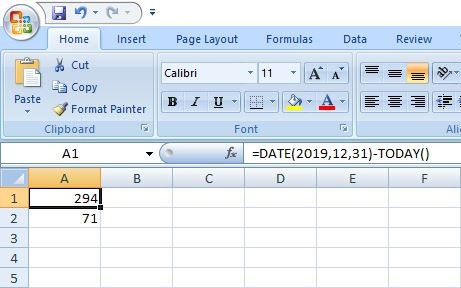And =TODAY()-DATE(2018,12,31) to find out days passed since last years new years eve.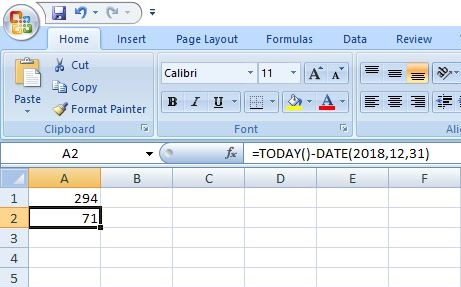By using TODAY function we input dates directly to a formula, so you can not do this for multiple dates at once. You have to manually input dates every time you want to find out days. Also, TODAY() is a volatile function which means the value will update itself when you open the spreadsheet on the next day.

### 5. Calculate Working Days Between Two Dates

To find out only working days between two dates you need to use the function NETWORKDAYS(). It is very similar to DATEDIF() function and you use arguments in the same manner. The formula for this is =NETWORKDAYS(start_date_cell, end_date_cell). In our example, start date lies in A2 and end date in B2 so the formula becomes =NETWORKDAYS(A2, B2).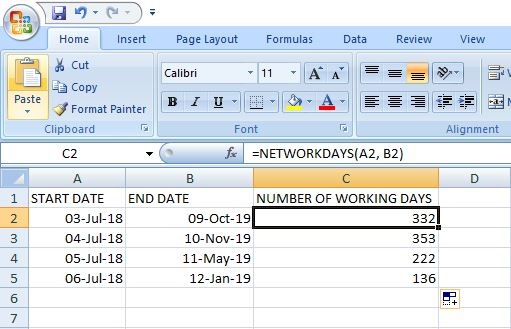Note: By default, Saturdays and Sundays are considered as weekends but you can also change them in Excel. In which you can specify which days to consider as holidays or not– working days.

There is also an option to manually enter a list of holidays in the cells and mention in the formula to leave out these days. For example, A8 and A9 contain the days you want to leave out from working days then the formula will be: =NETWORKDAYS(A2, B2, \$A\$8:\$A\$9). You add a third argument in the formula to leave out those days from the result.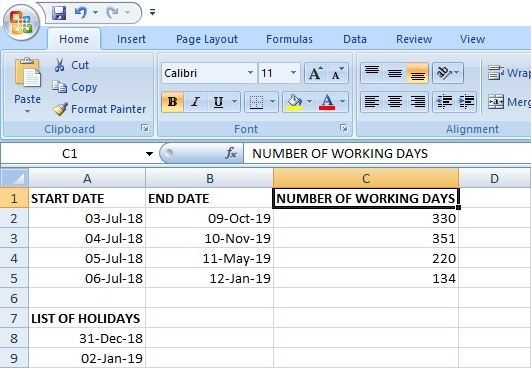Also Read: Best Scientific Calculator Apps

### Conclusion

This is it guys, these are the methods in Excel that are used to calculate the number of days between two dates. At some instances a function might not work in your sheet, we recommend you to try another. And if the results are not accurate, try to apply general format of dates in the cell. If you have any confusion and issue regarding any of these methods, please do ask us in the comments.

Amazon and the Amazon logo are trademarks of Amazon.com, Inc, or its affiliates.A laconic and introverted person, who is trying to blend in with normal people with his writings.